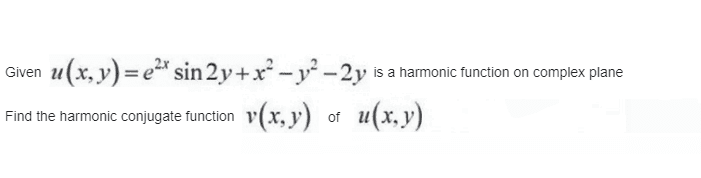# Harmonic function problem

• MHB
• jaychay
In summary, the conversation discusses how a harmonic conjugate function is related to the concept of holomorphic functions and complex differentiability. The next step in the process is to integrate the partial derivative of the harmonic conjugate function with respect to one of the variables, treating the other variable as a constant. This will result in a function that satisfies the necessary conditions for being a harmonic conjugate. f

#### jaychayI am really struggle with this question.

Hint: A harmonic conjugate function $v$ must have that $\frac{\partial u}{\partial x}=\frac{\partial v}{\partial y}$ and $\frac{\partial u}{\partial y}=-\frac{\partial v}{\partial x}$, so that the function given by $f(x+iy)=u(x,y)+iv(x,y)$ is holomorphic (complex differentiable).

Hint: A harmonic conjugate function $v$ must have that $\frac{\partial u}{\partial x}=\frac{\partial v}{\partial y}$ and $\frac{\partial u}{\partial y}=-\frac{\partial v}{\partial x}$, so that the function given by $f(x+iy)=u(x,y)+iv(x,y)$ is holomorphic (complex differentiable).
Can you tell what is the next step that I should do please ?

#### Attachments

Can you tell what is the next step that I should do please ?
It's a partial derivative (note the round d's). When we partially differentiate with respect to $x$, then that means that we treat $y$ as a constant.
More concisely:
$$\frac{\partial}{\partial x}(y)=0$$
So you can simplify your expression for $\frac{\partial u}{\partial x}$ a bit.

Next step is to integrate $\frac{\partial u}{\partial x}$ with respect to $y$ to find $v(x,y)$.
When we do so, we treat $x$ as a constant.

Let me give an example.
Suppose we have $u(x,y)=xy$. Then we have $\frac{\partial u}{\partial x}=y$.
And $v(x,y)=\int \frac{\partial u}{\partial x} \,dy = \int y\,dy = \frac 12y^2 + C(x)$, where $C(x)$ is some function of $x$.
We can verify by evaluating $\frac{\partial v}{\partial y} = \frac{\partial}{\partial y}\Big(\frac 12y^2 + C(x)\Big) = y$.
As we can see, we get indeed that $\frac{\partial u}{\partial x}=\frac{\partial v}{\partial y}$.

Last edited: